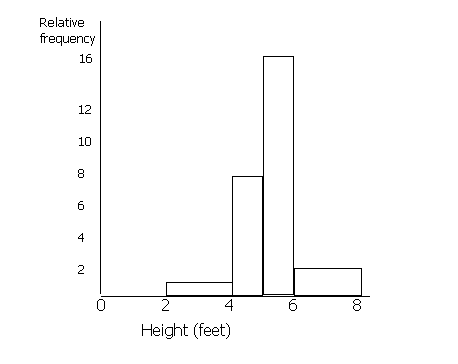## Histograms

Histograms are similar to bar charts apart from the consideration of areas. In a bar chart, all of the bars are the same width and the only thing that matters is the height of the bar. In a histogram, the area is the important thing.

Example

Draw a histogram for the following information.

 Height (feet): Frequency Relative Frequency 0-2 0 0 2-4 1 1 4-5 4 8 5-6 8 16 6-8 2 2

(Ignore relative frequency for now). It is difficult to draw a bar chart for this information, because the class divisions for the height are not the same. The height is grouped 0-2, 2-4 etc, but not all of the groups are the same size. For example the 4-5 group is smaller than the 0-2 group.

When drawing a histogram, the y-axis is labelled 'relative frequency' or 'frequency density'. You must work out the relative frequency before you can draw a histogram. To do this, first you must choose a standard width of the groups. Some of the heights are grouped into 2s (0-2, 2-4, 6-8) and some into 1s (4-5, 5-6). Most are 2s, so we shall call the standard width 2. To make the areas match, we must double the values for frequency which have a class division of 1 (since 1 is half of 2). Therefore the figures in the 4-5 and the 5-6 columns must be doubled. If any of the class divisions were 4 (for example if there was a 8-12 group), these figures would be halved. This is because the area of this 'bar' will be twice the standard width of 2 unless we half the frequency.

If you are having problems working out the height of each of the bars, you can use the formula

Area of bar = frequency x standard width